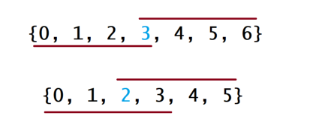03 多数元素

## 题解

### 方法一：哈希表

java实现

``````class Solution {
private Map<Integer, Integer> countNums(int[] nums) {
Map<Integer, Integer> counts = new HashMap<Integer, Integer>();
for (int num : nums) {
if (!counts.containsKey(num)) {
counts.put(num, 1);
} else {
counts.put(num, counts.get(num) + 1);
}
}
return counts;
}

public int majorityElement(int[] nums) {
Map<Integer, Integer> counts = countNums(nums);

Map.Entry<Integer, Integer> majorityEntry = null;
for (Map.Entry<Integer, Integer> entry : counts.entrySet()) {
if (majorityEntry == null || entry.getValue() > majorityEntry.getValue()) {
majorityEntry = entry;
}
}

return majorityEntry.getKey();
}
}
``````

python实现

``````
class Solution:
def majorityElement(self, nums: List[int]) -> int:
counts = collections.Counter(nums)
return max(counts.keys(), key=counts.get)``````

## 方法二：排序

### 思路java

``````class Solution {
public int majorityElement(int[] nums) {
Arrays.sort(nums);
return nums[nums.length / 2];
}
}``````

python

``````class Solution:
def majorityElement(self, nums: List[int]) -> int:
nums.sort()
return nums[len(nums) // 2]``````Lv1

helloworld开发者社区网站站长

89

43

98